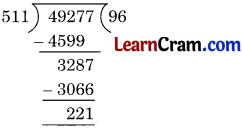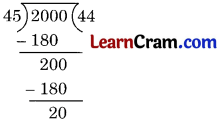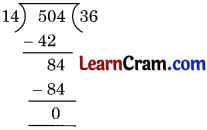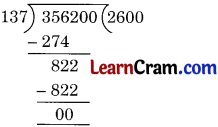# DAV Class 6 Maths Chapter 1 Worksheet 6 Solutions

The DAV Class 6 Maths Book Solutions Pdf and DAV Class 6 Maths Chapter 1 Worksheet 6 Solutions of Natural Numbers and Whole Numbers offer comprehensive answers to textbook questions.

## DAV Class 6 Maths Ch 1 WS 6 Solutions

Question 1.

(а) 2781 ÷ 35
Solution:
2781 ÷ 35Check:
Dividend = Quotient × Divisor + Remainder
2781 = 79 × 35 + 16 = 2765 + 16
Hence 2781 = 2781
Hence verified.

(b) 49277 ÷ 511
Solution:
49277 ÷ 511Check:
Dividend = Quotient × Divisor + Remainder
49277 = 511 × 96 + 221
= 49056 + 221
= 49277
Hence 49277 = 49277
Hence Verified.(c) 7335 ÷ 122
Solution:
7335 ÷ 122Check:
Dividend = Quotient × Divisor + Remainder
7335 = 0 × 122 + 15
= 7320 + 15
= 7335
Hence 7335 = 7335
Hence Verified.

(d) 64895 ÷ 247
Solution:
64895 ÷ 247Check:
Dividend = Quotient × Divisor + Remainder
64895 = 262 × 247 + 181
= 64714 + 181
= 64895
Hence 64895 = 64895
Hence Verified.Question 2.
Find the least number that should be subtracted from 1000 so that 35 divides the difference exactly.
Solution:
Dividing 1000 by 35Hence 20 is the required least number.

Question 3.
Find the least number that should be added to 2000 so that 45 divides the sum exactly.
Solution:
Dividing 2000 by 45∴ Required number = 45 – 20 = 25.Question 4.
Find the largest 5-digit number which is exactly divisible by 40.
Solution:
The largest 5-digit number = 99999
Dividing 99999 by 40∴ Required number = 99999 – 39 = 99960

Question 5.
In a parade, the soldiers are arranged in 14 rows. If the number of soldiers is 504, find the number of soldiers in each row.
Solution:
Dividing 504 by 14∴ Required number of soldiers = 36.Question 6.
In a dance class, 137 students got themselves enrolled. If the total fee collected is ₹ 356200, find the fee paid by each student.
Solution:
Dividing 356200 by 137Hence required amount paid by each student is ₹ 2600.